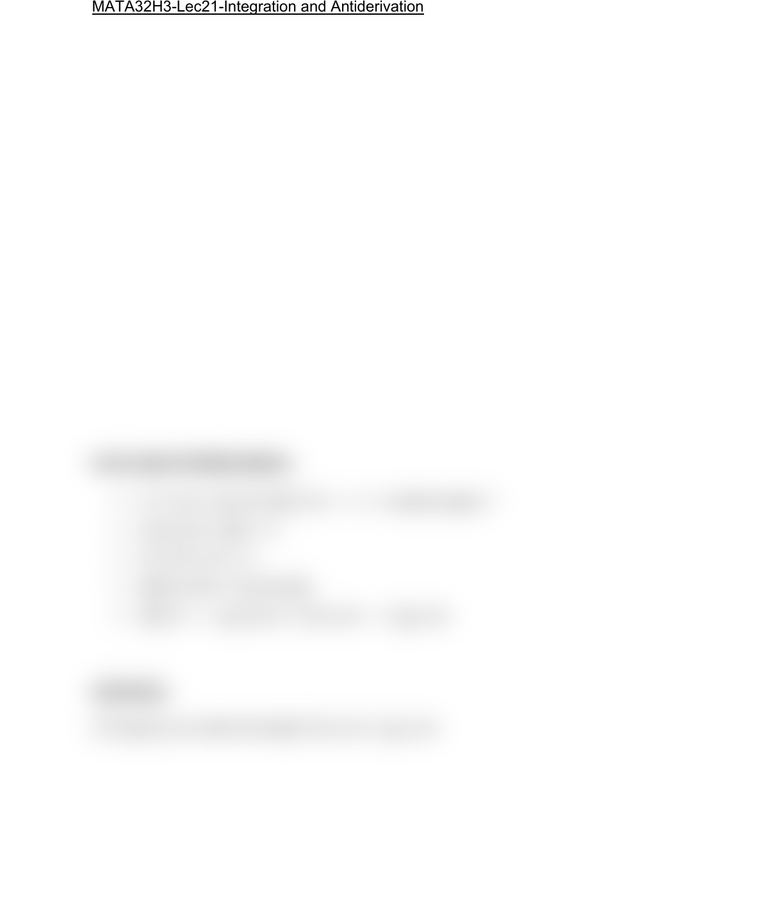Class Notes (1,100,000)
CA (640,000)
UTSC (30,000)
Mathematics (1,000)
MATA32H3 (200)
Lecture 21

# MATA32H3 Lecture 21: MATA32H3-Lec21-Integration and AntiderivationPremium

Department
Mathematics
Course Code
MATA32H3
Professor
Karimian Pour, C.
Lecture
21

This preview shows half of the first page. to view the full 2 pages of the document.MATA32H3-Lec21-Integration and Antiderivation
Integration Examples
1. f(x)= 5sqrt(x) = 5x^2
5x^(1/2)dx = 5 (3/2 x^(3/2)) + C
(10/3 x^(3/2) + C) = (10/3) (3/2 x^(1/2))
= 5x^(1/2)
2. (1/𝑥)dx = ln (x) + C
Reason: (d/dx) ln(x) + C = (1/x)
3. ln⁡(𝑥)dx = x ln(x) x + C
Check by differentiation
(d/dx) [x ln(x) x + C] = ln(x) + x(1/x) 1
= ln(x) + 1 1
= ln(x)
𝑥^𝑛 dx = ((x^(n+1))/(n+1)) + - C , n cannot equal -1
(1/𝑥) dx = ln(x) + C
𝑒^𝑡 dt = e^t + C
[𝑘𝑓(𝑥)dx] = k [𝑓(𝑥)dx]
[𝑓(𝑥)+⁡𝑔(𝑥)]⁡ 𝑑𝑥 = 𝑓(𝑥) dx + - 𝑔(𝑥) dx
WARNING:
𝑓(𝑥)𝑔(𝑥)]⁡ 𝑑𝑥 does not equal 𝑓(𝑥) dx 𝑔(𝑥) dx